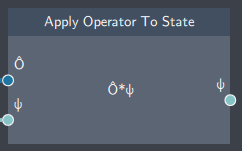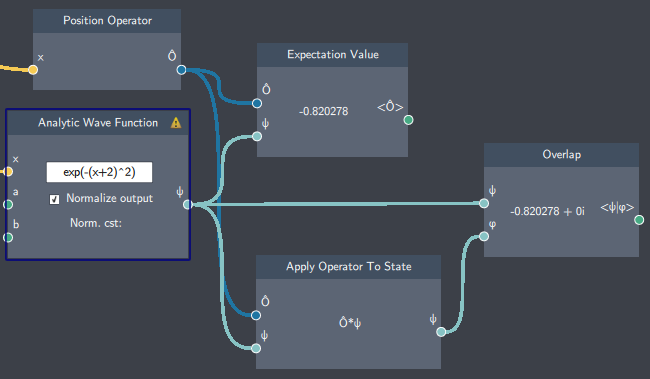# Apply Operator To State

## Description

The Apply Operator To State node applies an operator to a state. The operator applied could be any one of the operators such as $\hat{x}$, $\hat{p}$, $\hat{V}$ or $\hat{H}$.## Input

• Operator ($\hat{O}$): The operator that will be applied ($\hat{x}$, $\hat{p}$, $\hat{V}$ or $\hat{H}$).
• Wave Function ($\psi$): The state that the operator will be applied to.

## Content

The node takes a function as an input and gives a new function upon application of the operator.

## Output

• Wavefunction ($\psi$): The state after the operator has been applied.

## Example

In the example below, the Position Operator $\hat{x}$ is applied to a state, and then overlapped with the initial state ($\lt \psi | x \psi \gt$).

This essentially corresponds to finding the expectation value of $x$ ($\lt \psi | x | \psi \gt$) which is also shown below. It can be seen that the values from the Overlap and Expectation Value nodes are the same, which confirms the relationship between operator application and expectation values.